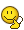# Double Slit: Separation and Path Length

• EmilyBergendahl
In summary, xbright is the amount of light coming through the slits, and d is the distance between the slits. The separation of the slits is 51.8 μm.

## Homework Statement

A double slit apparatus is held 1.2 m from a screen.
(a) When red light (λ = 600 nm) is sent through the double slit, the interference pattern on the screen shows a distance of 12.5 cm between the first and tenth dark fringes. What is the separation of the slits?
(b) What will be the difference in path length for the waves traveling from each slit to the tenth nodal line?​

## Homework Equations

(a) xbright = λLm / d
(b) path difference = (2m + 1)(λ / 2)

## The Attempt at a Solution

(a) xbright = λLm / d
d = λLm / xbright
d = (6.00 × 10-7 m)(1.2 m)(9) / 0.125 m
d = 5.18 × 10-5 m
d = 51.8 μm

(b) path difference = (2m + 1)(λ / 2)
path difference = [2(9) + 1][(6.00 × 10-7 m) / 2]
path difference = 5.7 × 10-6 m
path difference = 0.57 μm

Hi! I'm little bit confused on this optics review question. I ended up using the bright fringe formula instead of the dark fringe formula for part (a) and I want to make sure I didn't do any colossal damage in the process. I also want to make sure I used the right formula for part (b).

Thank you in advance!Hello again. This one can be most readily worked by counting along the adjacent bright spots. You need to know that the center bright spot (at ##\theta=0 ##) is m=0 so the bright spot to the left(i.e. towards the center) of dark spot #10 will be bright spot m=9. Again use ## m \lambda=d \sin{\theta} ## for the location of the bright spots. (This formula for the location of the primary maxima works for two slits, three slits, or even many many slits or lines ( spaced a distance "d" apart) like on a diffraction grating.)The rest should be straightforward. For part "b", first try to answer what the path difference distance must be from each of the two slits to the screen for the m=9 (constructive interference) bright spot. Then you should be able to figure out what the path difference needs to be to have destructive interference at a location directly adjacent to the bright spot. editing... reading through your solution. Part "a" you solved correctly, other than to be more accurate, your x/L is actually ## \tan{\theta} ## rather than ## \sin{\theta} ##. For part b, 5.7 E-6 m=5.7 ## \mu m ##

Last edited:
•EmilyBergendahl
I'm still a bit confused. Given the information in the problem, how would I go about finding theta?

## tan(\theta)=x/L ## where x=12.5 cm and L=120 cm (1.2 m). (you might need practice with trigonometry). ## \theta=arctan(12.5/120) ##. (You get an answer in degrees or radians. You then use your calculator to give you ## \sin{\theta} ##. The answer will be approximately 12.5/120 ,but this is more accurate. For a quick estimate ## \sin{\theta} ## is approximately ## \tan{\theta}=x/L ## but it's not exact for larger angles. (For this one, it may be an ok approximation). I edited post #2, so be sure and read the edited version.

Last edited:
•EmilyBergendahl
Oh, I see. I did the conversion to micrometers wrong... Part (b) should actually be 5.7 μm.

And for part (a), I recalculated using mλ = dsinθ, and I got 5.21 × 10-5 m, or 5.21 μm.

ok good. (Should read 52.1 ## \mu m ## for your last answer.) A very slight difference from 51.8 ##\mu m ##. Very good.

•EmilyBergendahl
Oh, man...Seeing all these decimal errors I'm realizing it's really time for me to sleep... Haha.

Thank you again for all your help Charles! You've been a lifesaver today!•